NCERT Class 9 Maths Constructions

# NCERT Class 9 Maths Constructions

This chapter 11 focuses on introduction and basic constructions like bisecting the angle, perpendicular bisector of the given line, angle of 60 with the given line and followed by constructions of triangle which include constructing a triangle given its base, a base angle and sum of other two sides, constructing a triangle given its base, a base angle and the difference of the other two sides and also constructing a triangle, given its perimeter and its two base angles. All the constructions are explained with different figures and diagrams.

## Chapter 11 Ex.11.1 Question 1

Construct an angle of $$90^\circ$$ at the initial point of a given ray and justify the construction.

### Solution

Reasoning:

We need to construct two adjacent angles each of $$60$$ degrees and bisect the second one to construct $$90$$ degree.

Steps of Construction: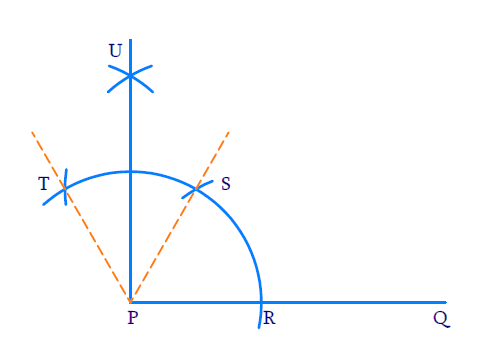(i) Draw ray $$PQ$$

(ii) To construct $$60^{\circ}$$ angle. Draw an arc of any radius with $$P$$ as center intersecting $$PQ$$ at $$R$$ with $$R$$ as center and same radius draw an arc intersecting the previous arc at $$S$$. \begin{align}\angle {SPQ}=60^{\circ}\end{align}

(iii) To construct adjacent $$60^{\circ}$$ angle. With $$S$$ as the center and same radius as before intersecting the initial arc at $$T$$ $$\angle {TPS}$$ will be $$60^\circ$$

(iv) To bisect $$\angle {TPS} :$$

With $$T$$ and $$S$$ as centers and same radius as before draw two arcs to intersect each other at $$U$$. \begin{align}\angle {UPS}=\frac{1}{2} \angle {TPS}=30^{\circ}\end{align}

(v)  Join $$P$$ and $$U$$ to get an angle of $$60^{0}$$ at initial point $$P$$.

\begin{align} \angle {UPQ} &=\angle {UPS}+\angle {SPR} \\ &=30^{\circ}+60^{\circ} \\ &=90^{\circ} \end{align}

## Chapter 11 Ex.11.1 Question 2

Construct an angle of $$45^\circ$$ at the initial point of a given ray and justify the construction.

### Solution

Reasoning:

We need to construct two adjacent angles each of $$60^\circ$$ degrees and bisect the second one to construct $$90^\circ$$ . Then bisect the $$90^\circ$$ angle to get $$45^\circ$$

\begin{align}\frac{60^{0}+\frac{60^{0}}{2}}{2}=45^{0}\end{align}

Steps of Construction: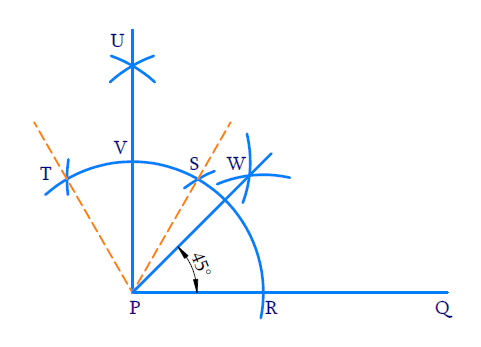(i) Draw ray $$PQ$$.

(ii) To construct an angle of $$60^{o}$$ . With $$P$$ as center draw a wide arc of any radius to intersect the ray at $$R$$. With $$R$$ as center and same radius draw an arc to intersect the initial one at $$S$$. $$\angle {SPR}=60^{\circ}$$ .

(iii) To construct adjacent angle of $$60^{\circ}$$ .

With $$S$$ as centre and same radius draw an arc to intersect the previous arc at $$T$$

$\angle {TPS}=60^{\circ}$

(iv) To bisect $$\angle {TPS}$$

With $$T$$ and $$S$$ as centre and same radius, draw arcs to intersect each other at $$U$$.

\begin{align}\angle {UPS}=\frac{1}{2} \angle {TPS}=30^{\circ}\end{align}

(v) Join $$P$$ and $$U$$ to intersect the initial arc at $$V$$.

\begin{align} \angle {UPQ} &=\angle {UPS}+\angle {SPR} \\ &=30^{\circ}+60^{\circ} \\ &=90^{\circ} \end{align}

(vi) To bisect $$\angle {UPQ}$$

With $$R$$ and $$V$$ as centers and radius greater than half of $$RV$$, draw arc to intersect each other at $$W$$. Join $$PW$$. $$PW$$ is the angle bisector of $$\angle U P Q$$

\begin{align} \angle {WPQ} &=\frac{1}{2} \angle {UPQ} \\ &=\frac{1}{2} \times 90^{\circ} \\ &=45^{\circ} \end{align}

(vii) Ray $$PW$$ forms an angle of $$45^{\circ}$$ with ray $$PQ$$ at the initial point.

## Chapter 11 Ex.11.1 Question 3

Construct the angles of the following measurements:

(i) $$30^{\circ}$$

(ii) $$22 \frac{1}{2}^{\circ}$$

(iii) $$15^{\circ}$$

### Solution

$${\rm{(i)}}\;\;30^{\circ}$$

Reasoning:

We need to construct an angle of $$60$$ degrees and then bisect it to get an angle measuring $$30^\circ$$

Steps of Construction: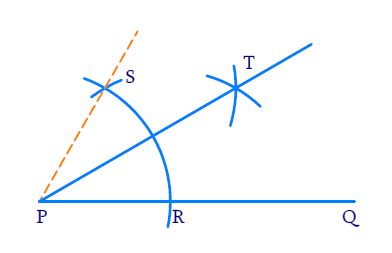(i) Draw ray $$PQ$$.

(ii) To construct an angle of $$60^{\circ}$$ .

With $$P$$ as centre and any radius, draw a wide arc to intersect $$PQ$$ at $$R$$. With $$R$$ as centre and same radius draw an arc to intersect the initial arc at $$S$$. $$\angle {SPR}=60^{\circ}$$

(iii) To bisect $$\angle {SPR}$$

With $$R$$ and $$S$$ as centres and same radius draw two arcs to intersect at $$T$$. Join $$P$$ and $$T$$ i.e. $$PT$$ is the angle bisector. Hence,

\begin{align}\angle {TPR}=\frac{1}{2} \angle {SPR}=30^{\circ}\end{align}

$${\rm{(ii)}}\;\;22 \frac{1}{2}^{\circ}$$

Reasoning:

We need to construct two adjacent angles of and bisect the second one to get a angle. This has to be bisected again to get a $$45^\circ$$ angle. The $$45^\circ$$ angle has to be further bisected to get \begin{align}22 \frac{1}{2}^{\circ}\end{align} angle.

\begin{align} 22 \frac{1}{2}^{\circ} &=\frac{45^{\circ}}{2} \\ 45^{\circ} &=\frac{90^{\circ}}{2}=\frac{30^{\circ}+60^{\circ}}{2} \end{align}

Steps of Construction: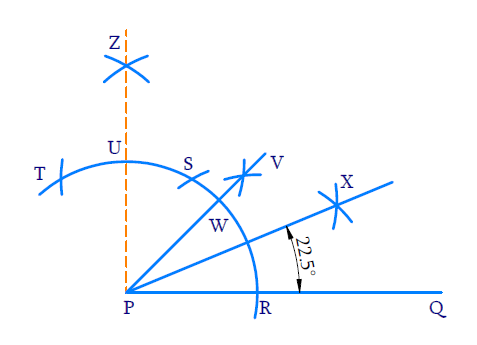(i) Draw ray $$PQ$$

(ii) To construct an angle of $$60^{\circ}$$

With $$P$$ as center and any radius draw a wide arc to intersect $$PQ$$ at $$R$$. With $$R$$ as center and same radius draw an arc to intersect the initial arc at $$S$$. $$\angle {SPR}=60^{\circ}$$

(iii)To construct adjacent angle of $$60^{\circ}$$ .

With $$S$$ as the center and same radius as before, draw an arc to intersect the initial arc at $$T$$ $$\angle {TPS}=60^{\circ}$$ .

(iv)To bisect $$\angle {TPS}$$

With $$T$$ and $$S$$ as centers and same radius as before, draw arcs to intersect each other at $$Z$$ Join $$P$$ and $$Z$$ $$\angle {ZPQ}=90^{\circ}$$

(v) To bisect $$\angle {ZPQ}$$

With $$R$$ and $$U$$ as centers and radius than half of $$RU$$, draw arcs to intersect each other at $$V$$. Join $$P$$ and $$V$$. $$\angle {VPQ}=45^{0}$$

(vi) To bisect $$\angle {VPQ}=45^{0}$$

With $$W$$ and $$R$$ as centers and radius greater than half of $$WR$$, draw arcs to intersect each other at $$X$$. Join $$P$$ and $$X$$. $$PX$$ bisects $$\angle {VPQ}$$

Hence,

\begin{align} \angle {XPQ} &=\frac{1}{2} \angle {WPQ} \\ &=\frac{1}{2} \times 45^{0} \\ &=22 \frac{1}{2} \end{align}

$${\rm{(iii)}}\;\;15^{\circ}$$

Reasoning:

We need to construct an angle of 60 degrees and then bisect it to get an angle measuring $$30^\circ$$. This has to be bisected again to get a $$15^\circ$$ angle.

\begin{align}15^{0}=\frac{30^{\circ}}{2}=\frac{\frac{60^{0}}{2}}{2}\end{align}

Steps of Construction: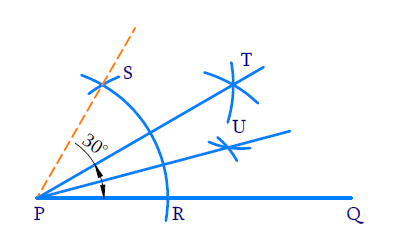(i) Draw ray $$PQ$$.

(ii) To construct an angle of $$60^{\circ}$$ .

With $$P$$ as center and any radius draw a wide arc to intersect $$PQ$$ at $$R$$. With $$R$$ as center and same radius draw an arc to intersect the initial arc at $$S$$. $$\angle {SPR}=60^{\circ}$$

(iii) Bisect $$\angle {SPR}$$ . With $$R$$ and $$S$$ as centers and radius greater than half of $$RS$$ draw arcs to intersect each other at $$T$$. Join $$P$$ and $$T$$ i.e. $$PT$$ is the angle bisector of $$\angle {SPR}$$ .

\begin{align} \angle {TPQ} &=\frac{1}{2} \angle{SPR} \\ &=\frac{1}{2} \times 60^{\circ} \\ &=30^{\circ} \end{align}

(iv)To bisect $$\angle {TPQ}$$

With $$R$$ and $$W$$ as centers and radius greater than half of $$RT$$, draw arcs to intersect each other at $$U$$ Join $$P$$ and $$U$$. $$PU$$ is the angle bisector of $$\angle {TPQ}$$ .

\begin{align}\angle {UPQ}=\frac{1}{2} \angle {TPQ}=15^{\circ}\end{align}

## Chapter 11 Ex.11.1 Question 4

Construct the following angles and verify by measuring them by a protractor:

(i) $$75^{\circ}$$

(ii) $$105^{\circ}$$

(iii) $$135^{\circ}$$

### Solution

$${\rm{(i)}}\;\;75^{\circ}$$

Reasoning:

We need to construct two adjacent angles of $$60^{\circ}$$.The second angle should be bisected twice to get a $$15^{\circ}$$ angle.

\begin{align}75^{\circ}&=60^{\circ}+15^{\circ} \\ 15^{\circ}&=\frac{30^{\circ}}{2}=\frac{60^{\circ}}{2} \div \frac{1}{2}\end{align}

Steps of Construction: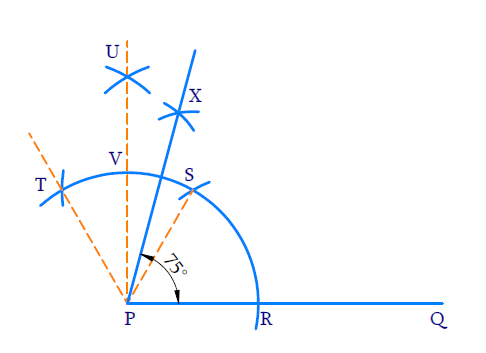(i) Draw ray $$PQ$$.

(ii) To construct an angle of $$60^{\circ}$$ .

With $$P$$ as centre and any radius draw a wide arc to intersect $$PQ$$ at $$R$$ With $$R$$ as centre and same radius draw an arc to intersect the initial arc at $$S$$ .$$\angle \mathrm{SPR}=60^{\circ}$$

(iii) To construct adjacent angle of $$60^{\circ}$$ with $$S$$ as center and same radius draw an arc to $$T$$ intersect the initial arc.

(iv) To bisect $$\angle \mathrm{SPT}$$

With $$T$$ and $$S$$ as centres and same radius draw arcs to bisect each other at $$U$$ Join $$U$$ and $$P$$. \begin{align}\angle \mathrm{UPS}=\frac{1}{2} \angle \mathrm{TPS}=30^{\circ}\end{align}

(v) To bisect $$\angle \mathrm{UPS}$$

With $$V$$ and $$S$$ as centers and radius greater than half of $$VS$$ draw arcs to intersect each other at $$X$$

\begin{align} \angle {XPS} &=\frac{1}{2} \angle {UPS} \\ &=\frac{1}{2} \times 30^{0} \\ &=15^{\circ} \\ \angle {XPQ} &=\angle {XPS}+\angle {SPQ} \\ &=15^{\circ}+60^{\circ} \end{align}

$${\rm{(ii)}}\;\;105^{\circ}$$

Reasoning:

We need to construct two adjacent angles of $$60^{\circ}$$ . In the second angle we need to bisect it to get two $$30^{\circ}$$ angles. The second $$30^{\circ}$$ angle should be bisected again to get a $$15^{\circ}$$ angle. Together we can make an angle of $$105^{\circ}$$ .

\begin{align} 105^{\circ} &=60^{\circ}+45^{\circ} \\ 45^{\circ} &=60^{\circ}+30^{\circ}+15^{\circ} \end{align}

Steps of Construction: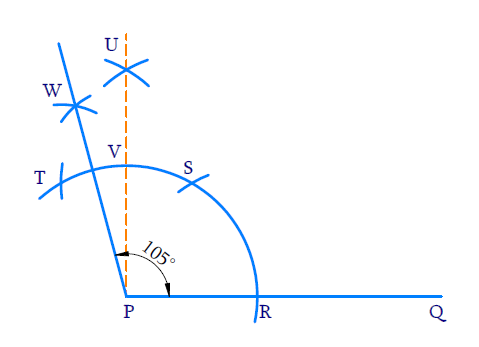(i) Draw ray $$PQ$$

(ii) To construct an angle of $$60^{\circ}$$.

With $$P$$ as centre and any radius draw a wide arc to intersect $$PQ$$ at $$R$$. With $$R$$ as centre and same radius draw an arc to intersect the initial arc at $$S$$. $$\angle \mathrm{SPR}=60^{\circ}$$

(iii) To construct an adjacent angle of $$60^{\circ}$$ with $$S$$ as the center and same radius as before draw an arc to intersect the initial arc at $$T$$. $$\angle \mathrm{TPS}=60^{\circ}$$

(iv) To bisect $$\angle \mathrm{TPS}$$

With $$T$$ and $$S$$ as centres and same radius draw arcs to bisect each other at $$U$$. Join $$U$$ and $$P$$

\begin{align}\angle \text{UPS = }\frac{1}{2}\angle \text{TPS }=\frac{1}{2}\times {{60}^{0}}\text{=30}{}^\circ \end{align}

(v)To bisect $$\angle \text{UPT}$$

With $$T$$ and $$V$$ as centers and radius greater than half of $$TV,$$draw arcs to intersect each other at $$W$$. Join $$P$$ and $$W$$.

\begin{align} \angle {WPU} &=\frac{1}{2} \angle {UTP} \\ &=\frac{1}{2} \times 30^{\circ} \\ &=15^{\circ} \\ \angle {WPR} &=\angle {WPU}+\angle {UPS}+\angle {SPR} \\ &=15^{\circ}+30^{\circ}+60^{\circ} \\ &=105^{\circ} \end{align}

$${\rm{(iii)}}\;\;135^{\circ}$$

Reasoning:

We need to construct three adjacent angles of $$60^{\circ}$$ each. The third angle should be bisected twice successively to get an angle of $$15^{\circ}$$ . Together we will get an angle of $$135^{\circ}$$ .

\begin{align} 135^{\circ} &=15^{\circ}+60^{\circ}+60^{\circ} \\ 15^{\circ} &=\frac{60^{\circ}}{2} \div \frac{1}{2} \end{align}

Steps of Construction: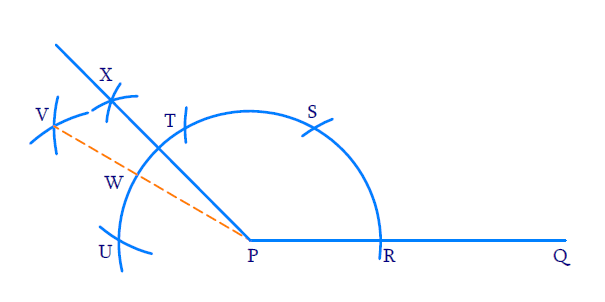(i) Draw ray$$PQ$$

(ii) To construct an angle of $$60^{\circ}$$ .

With $$P$$ as the center and any radius draw an arc to intersect $$PQ$$ at $$R$$.$$\angle \mathrm{SPR}=60^{\circ}$$

(iii) To construct adjacent angle of $$60^{\circ}$$

With $$S$$ as the center and same radius as before draw an arc to intersect the initial arc at $$T$$. $$\angle \mathrm{TPS}=60^{\circ}$$

(iv) To construct the second adjacent angle of $$60^{\circ}$$

With $$T$$ as centre and some radius as before draw an arc to intersect the initial arc at $$U$$

$\angle \mathrm{UPT}=60^{\circ}$

(v) To bisect $$\angle \mathrm{UPT}$$

With $$T$$ and $$U$$ as centers and same radius as before draw an arc to intersect each other at $$V$$ $$\angle \mathrm{VPT}=\angle \mathrm{VPU}=30^{\circ}$$

(vi) To bisect $$\angle \mathrm{VPT}$$

With $$W$$ and $$T$$ as centers and radius greater than half of $$WT$$ draw arcs to intersect each other at $$X$$.

\begin{align}XPT&=\angle XPV \\&=7\frac{1}{2}\angle V\text{PT}\\&=\frac{1}{2}\times {{30}^\circ}=\,15{}^\circ \\ \\ \angle XPQ &= \angle \text{XPT + }\angle TPS + \angle SPR \\&= 15{}^\circ + 60{}^\circ + 60{}^\circ \\&=135{}^\circ \,\,\, \\\end{align}

## Chapter 11 Ex.11.1 Question 5

Construct an equilateral triangle, given its side and justify the construction.

### Solution

Reasoning:

An equilateral triangle has three equal sides and three angles equal to $$60^\circ$$.We know how to construct an angle of $$60^{\circ}$$ .

Steps of Construction: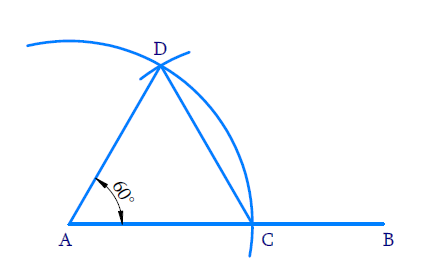(i) Draw ray $$AB$$

(ii) With $$A$$ as center and radius equal to $$3\;\rm{cm}$$ draw an arc to cut ray $$AB$$ at $$C$$ such that $$AC= 3\;\rm{cm}$$

(iii) With $$C$$ as the center and radius equal to $$AC$$, draw an arc to intersect the initial arc at $$D$$.

(iv) Join $$AD$$ and $$AC$$.

Thus,Triangle $$ADC$$ is an equilateral triangle.

Justification:

$$\rm{AC = AD}$$ (By construction since the radius of the arc is the same)

$$\rm{AC = CD}$$  (By construction since the same radius was used again)

$$\therefore \rm{AC = AD = CD}$$

$$\therefore ADC$$ is an equilateral triangle

Constructions | NCERT Solutions
Instant doubt clearing with Cuemath Advanced Math Program